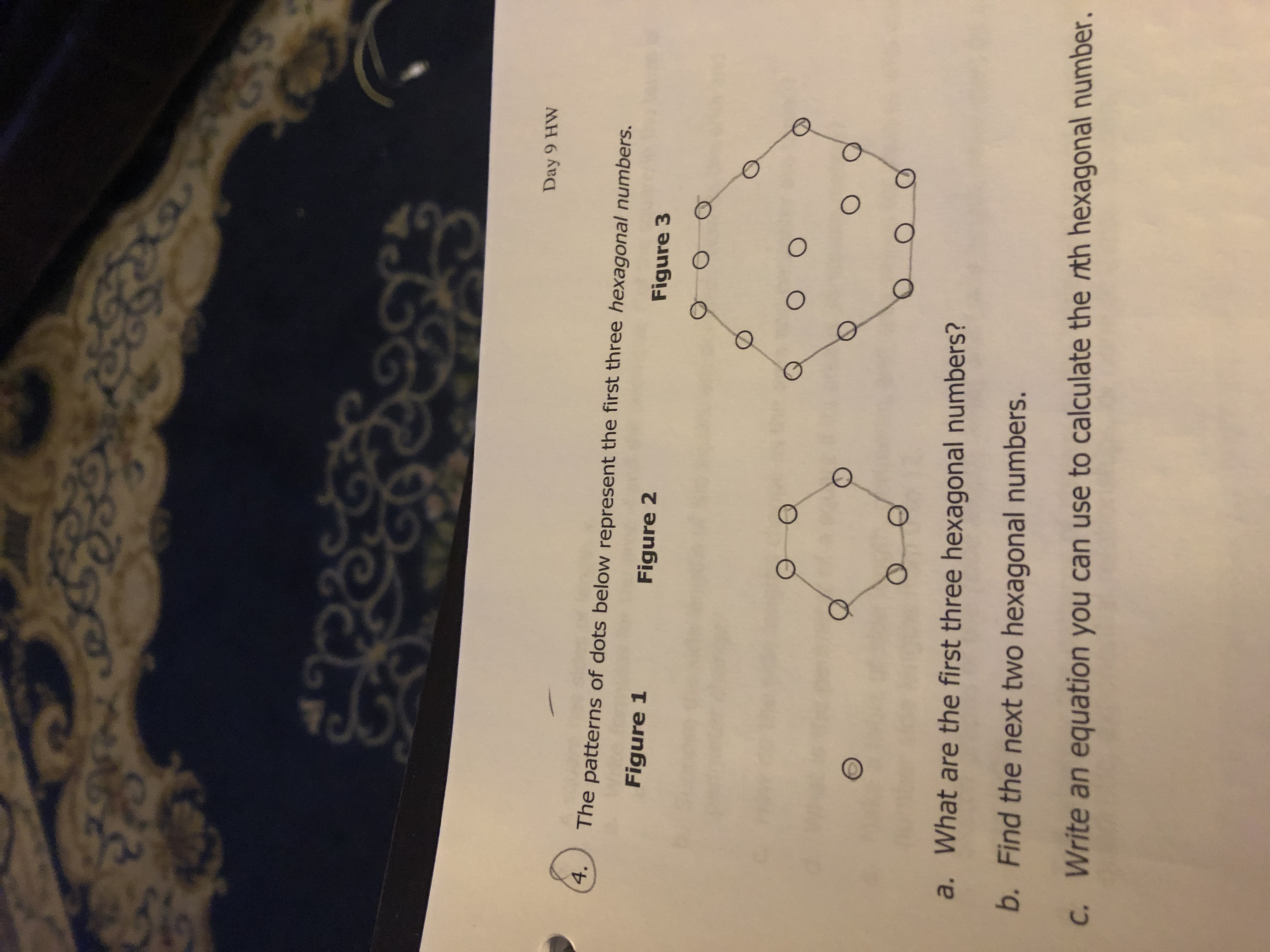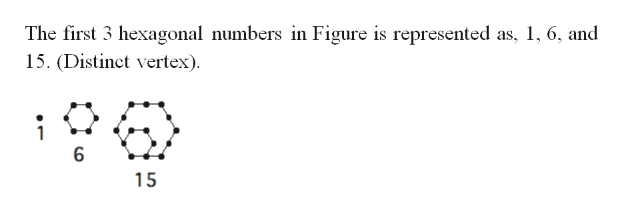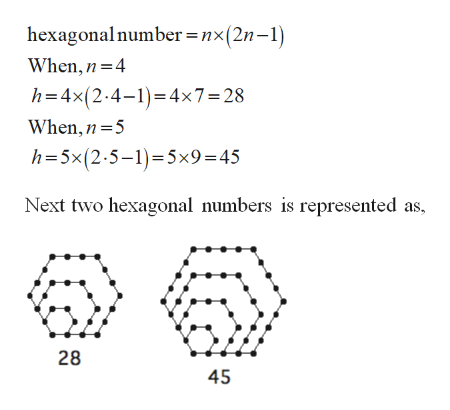# 2C%GD90Day 9 HW4.The patterns of dots below represent the first three hexagonal numbers.Figure 1Figure 2Figure 3O Oa. What are the first three hexagonal numbers?b. Find the next two hexagonal numbers.C. Write an equation you can use to calculate the rith hexagonal number.

Question
22 viewshelp_outlineImage Transcriptionclose2 C %GD 90 Day 9 HW 4. The patterns of dots below represent the first three hexagonal numbers. Figure 1 Figure 2 Figure 3 O O a. What are the first three hexagonal numbers? b. Find the next two hexagonal numbers. C. Write an equation you can use to calculate the rith hexagonal number. fullscreen
check_circle

Step 1

Part (a):

The hexagon with n regularly spaced points on a side will be the number of points.help_outlineImage TranscriptioncloseThe first 3 hexagonal numbers in Figure is represented as, 1 6, and 15. (Distinct vertex) 6 15 fullscreen
Step 2

Part (b):                 &n...help_outlineImage Transcriptionclosehexagonalnumber =nx(2n-1) When,n 4 h=4x(2.4-14x7 28 When, n 5 h-5x(2-5-1 5x9=45 Next two hexagonal numbers is represented as 28 45 fullscreen

### Want to see the full answer?

See Solution

#### Want to see this answer and more?

Solutions are written by subject experts who are available 24/7. Questions are typically answered within 1 hour.*

See Solution
*Response times may vary by subject and question.
Tagged in

### Other